### Home > MC2 > Chapter 6 > Lesson 6.2.6 > Problem6-124

6-124.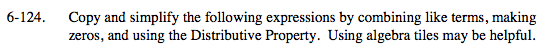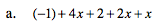Group like terms so that they can be combined.
(4x + 2x + x) + (−1 + 2)

7x + 1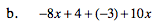Visualize 8 negative x tiles, 4 positive unit tiles, 3 negative unit tiles, and 10 positive x tiles. If a negative and a positive tile of the same value make a zero, how many tiles are remaining?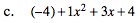Group like terms.
(−4 + 4) + 1x2 + 3x

Combine like terms.
0 + 1x2 + 3x

x2 + 3x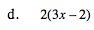This is 2 groups of (3x − 2). Visualize what that would look like with tiles.

Use the eTool below for help with this problem.
Click the link at right for the full version of the eTool: MC2 6-124 HW eTool